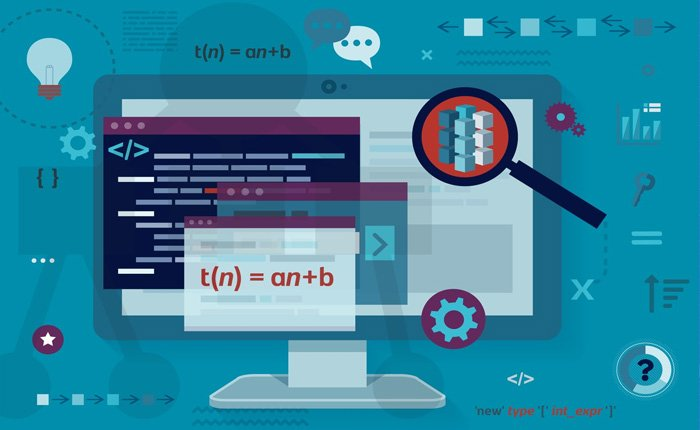# Certified in data structure

Wishlist Share
Share Course
Share On Social MediaCovers the design, analysis, and implementation of data structures and algorithms to solve engineering problems using an object‐oriented programming language.

Topics include elementary data structures,(including arrays, stacks, queues, and lists), advanced data structures (including trees and graphs), the algorithms used to manipulate these structures, and their application to solving practical engineering problems.

### What Will You Learn?

• Based on satisfactory completion of the course, a student should be able to:
• Formulate and apply object‐oriented programming, using C++, as a modern tool to solve engineering problems.
• Demonstrate an understanding of basic data structures (such as an array‐based list, linked list, stack, queue, binary search tree) and algorithms.
• ⦁ Demonstrate the ability to analyze, design, apply and use data structures and algorithms to solve engineering problems and evaluate their solutions.
•
• ⦁ Demonstrate an understanding of analysis of algorithms. Study an algorithm or program code segment that contains iterative constructs and analyze the asymptotic time complexity of the  algorithm or code segment.

### Course Content

#### Exam